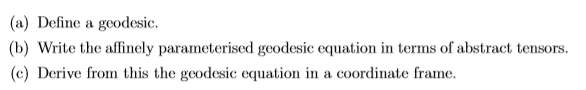# General Relativity - geodesic - affine parameter

• binbagsss

## Homework Statement

Question attached:see below

## The Attempt at a Solution

[/B]
my main question really is

1) what is meant by 'abstract tensors' as I have this for my definition:
to part a)
##V^u\nabla_uV^a=0##

but you do say that ##V^u=/dot{x^u}## ; x^u is a coordinate so, probably a stupid question, how is it really an 'abstract tensor'? I would say ##\nabla_u## is but not ##V^u##.

and

b) how this definition specifies/relies on being affinely parameterised. I have the definition as the tangent vector is parallel transported along itself. I would have thought that any tangent vector of a geodesic is parallel transported along itself- does it need to be affinely parameterised and what does this mean?

(I have seen the terminology 'affine connection' used elsewhere and that the Levi-Civita connection is one such connection, which is assumed in GR via the fundamental theorem of Riemann geometry - I posted a thread the other week on showing that definitions of geodesics agree due to this (the above and definition based on calculus of variations), and but in our lecture notes we have only defined a affine parameter as one such that dL/ds =0 , where L is the Lagrangian of a free-ly falling particle, we have not talked about affine connections and so I don't understand the definition of affine parameters in the context of parallel-transported tangent vector definition of geodesic as given above)- I guess the terms affine parameter and affine connection are related and this may cover it?

part b)

simply expand this out to get
##\ddot{x^u}+\Gamma_{ab}^{u}\dot{x^a}\dot{x^b}##

many thanks